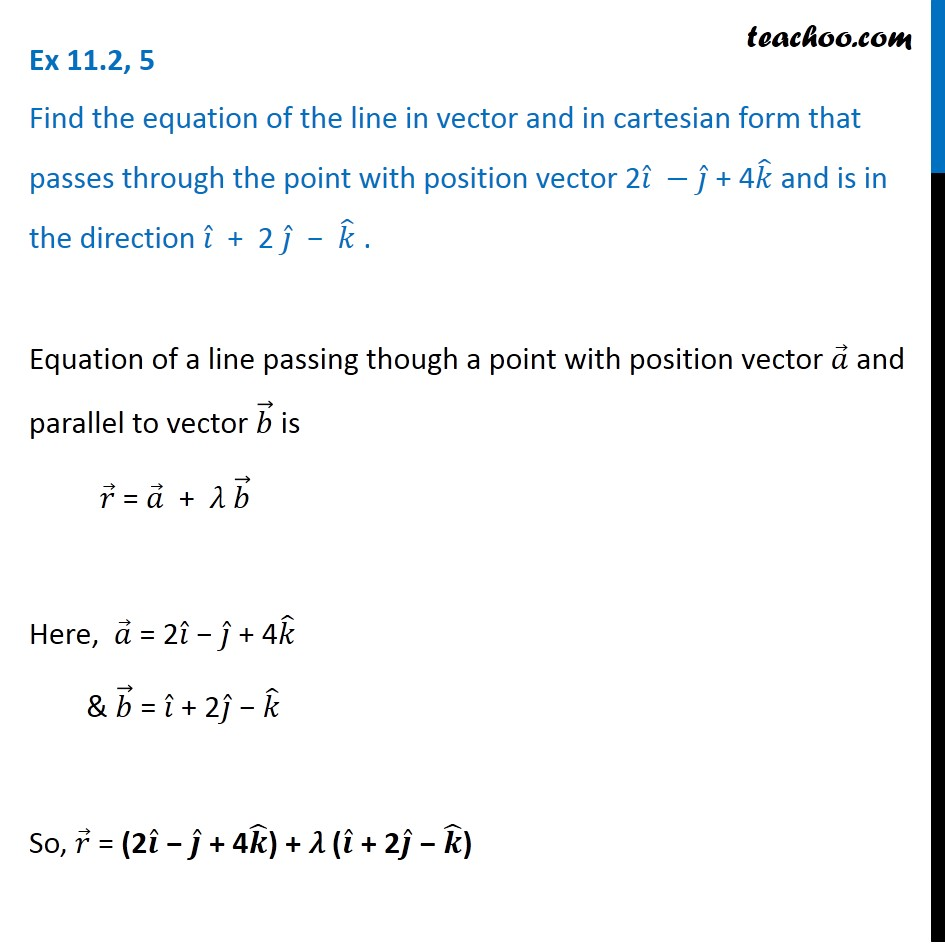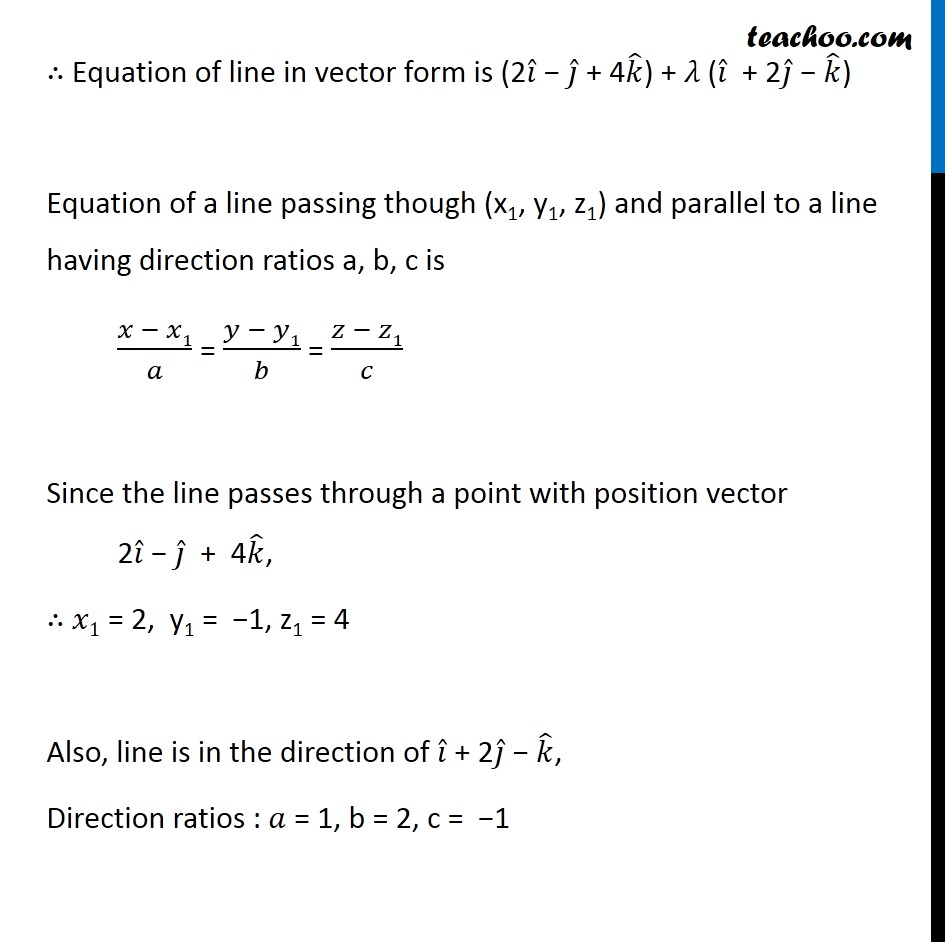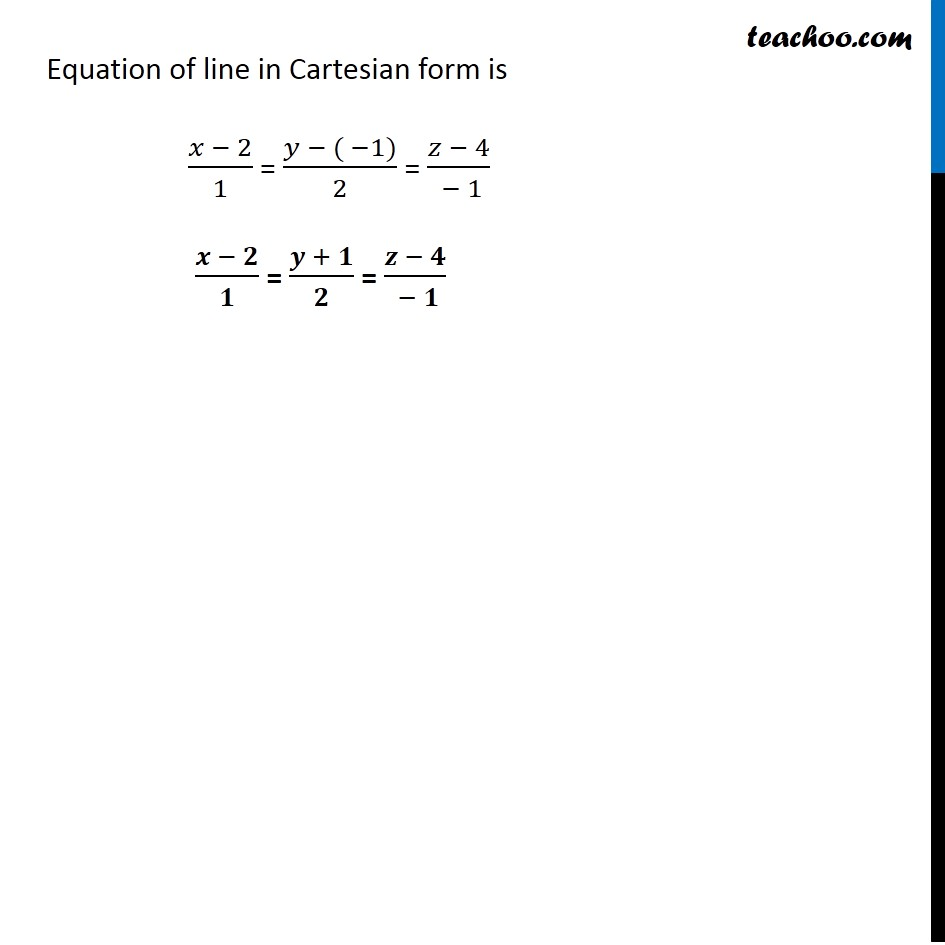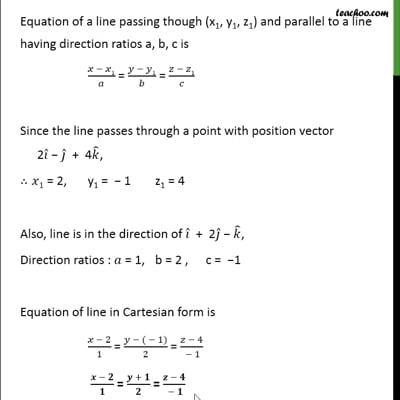Ex 11.2

Chapter 11 Class 12 Three Dimensional Geometry
Serial order wiseThis video is only available for Teachoo black users

Get live Maths 1-on-1 Classs - Class 6 to 12

### Transcript

Ex 11.2, 5 Find the equation of the line in vector and in cartesian form that passes through the point with position vector 2𝑖 ̂ − 𝑗 ̂ + 4𝑘 ̂ and is in the direction 𝑖 ̂ + 2 𝑗 ̂ − 𝑘 ̂ . Equation of a line passing though a point with position vector 𝑎 ⃗ and parallel to vector 𝑏 ⃗ is 𝑟 ⃗ = 𝑎 ⃗ + 𝜆 𝑏 ⃗ Here, 𝑎 ⃗ = 2𝑖 ̂ − 𝑗 ̂ + 4𝑘 ̂ & 𝑏 ⃗ = 𝑖 ̂ + 2𝑗 ̂ − 𝑘 ̂ So, 𝑟 ⃗ = (2𝒊 ̂ − 𝒋 ̂ + 4𝒌 ̂) + 𝜆 (𝒊 ̂ + 2𝒋 ̂ − 𝒌 ̂) ∴ Equation of line in vector form is (2𝑖 ̂ − 𝑗 ̂ + 4𝑘 ̂) + 𝜆 (𝑖 ̂ + 2𝑗 ̂ − 𝑘 ̂) Equation of a line passing though (x1, y1, z1) and parallel to a line having direction ratios a, b, c is (𝑥 − 𝑥1)/𝑎 = (𝑦 − 𝑦1)/𝑏 = (𝑧 − 𝑧1)/𝑐 Since the line passes through a point with position vector 2𝑖 ̂ − 𝑗 ̂ + 4𝑘 ̂, ∴ 𝑥1 = 2, y1 = −1, z1 = 4 Also, line is in the direction of 𝑖 ̂ + 2𝑗 ̂ − 𝑘 ̂, Direction ratios : 𝑎 = 1, b = 2, c = −1 Equation of line in Cartesian form is (𝑥 − 2)/1 = (𝑦 − ( −1))/2 = (𝑧 − 4)/( − 1) (𝒙 − 𝟐)/𝟏 = (𝒚 + 𝟏)/𝟐 = (𝒛 − 𝟒)/( − 𝟏)